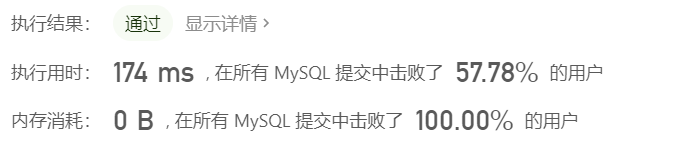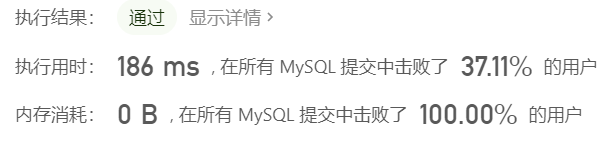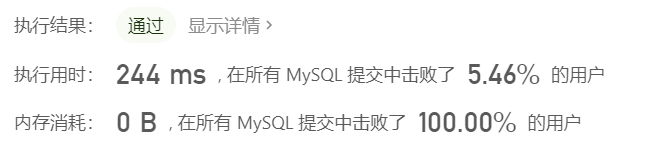## 627. 变更性别

给定一个 salary 表，如下所示，有 m = 男性 和 f = 女性 的值。交换所有的 f 和 m 值（例如，将所有 f 值更改为 m，反之亦然）。要求只使用一个更新（Update）语句，并且没有中间的临时表。

# 解法一：case 用法

语法：

``````case when <判断表达式> then <表达式>
when <判断表达式> then <表达式>
...
else <表达式>
end
``````

答案1：

``````update salary set
sex = case sex when 'm' then 'f'
else 'm'
end;
``````

答案2：

``````update salary set
sex = case when sex='m' then 'f'
else 'm'
end;
``````# 解法二：ASCII 转换

``````update salary set
sex = char(ascii('m') + ascii('f') - ascii(sex));
``````# 题解三：IF

``````update salary set sex = IF(sex = 'm', 'f', 'm')
``````